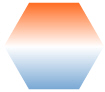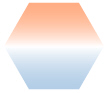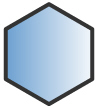You are viewing documentation for an older version. For current documentation - click here.

Here, we will discuss the polygon annotation and its properties. Here is how a polygon with 4 sides looks:The XML/JSON to create the above polygon is:

```<chart>
...
<annotations>
<annotationGroup id='Grp1' x='200' y='200' >
<annotation type='polygon' sides='4' color='639ACE' x='0' y='0' radius='50'/>
</annotationGroup>
</annotations>
...
</chart>```
```{
"chart": {},
...
"annotations": {
"groups": [
{
"id": "Grp1",
"x": "200",
"y": "200",
"items": [
{
"type": "polygon",
"sides": "4",
"color": "639ACE",
"x": "0",
"y": "0",
}
]
}
]
...
}
}```

As it is evident from the code above, we need to set type as polygon, define the number of sides, color and the starting x and y co-ordinates in the <annotation> element to build our polygon.

Setting the number of sides

We can have polygons with any number of sides. A polygon with 6 sides will look as under:Now, let's create a gradient background for the polygon. For that, all we need to do is add more colors to the color attribute. Here we will set color='639ACE,FFFFFF' to get the following effect.We can add as many colors as we want to the gradient. With color='639ACE,FFFFFF,FF5904', the polygon will look like:Now let's change the gradient angle. The same can be done using the fillAngle attribute. fillAngle='90' gives:We can also set the ratio in which the constituent colors of the gradient will be divided using the fillRatio attribute. Since we have 3 colors in the gradient here, we need 3 values in fillRatio, which should sum up to 100. If you like orange, then fillRatio='20,10,70' will look good to you:If you need to set the alpha (transparency) of the constituent colors of the gradient, then you have to use the fillAlpha attribute. Again this attribute should have as many values as there are colors in the gradient. This example consists of three gradient colors, one of the various combinations could be fillAlpha='50, 100, 50':Setting the start angle

When using polygons, many number of times we need to define the starting angle of the polygon. The same can be done using the startAngle attribute. Here we set startAngle='90' to get the following:Customizing polygon border

We can also add a border to the polygon by setting showBorder='1'. The polygon will dress up to be:The border color and thickness can be customized using the borderColor and borderThickness attributes. Setting borderColor='333333' and borderThickness='4' will give:So the final XML/JSON for the above polygon will look as under:

```<chart>
...
<annotations>
<annotationGroup id='Grp1'  x='200' y='200'>
<annotation type='polygon' sides='6' color='639ACE,FFFFFF' x='0' y='0' radius='50' startAngle='90'
showBorder='1' borderColor='333333' borderThickness='4'/>
</annotationGroup>
</annotations>
...
</chart>```
```{
"chart": {},
...
"annotations": {
"groups": [
{
"id": "Grp1",
"x": "200",
"y": "200",
"items": [
{
"type": "polygon",
"sides": "6",
"color": "639ACE,FFFFFF",
"x": "0",
"y": "0",
"startangle": "90",
"showborder": "1",
"bordercolor": "333333",
"borderthickness": "4"
}
]
}
]
...
}
}```

The various attributes for the polygon annotation using which it can be customized are: